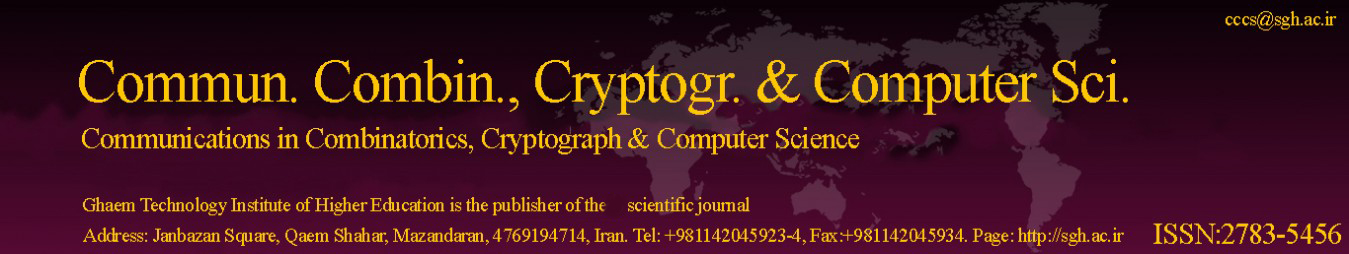Original Research Article

Article volume = 2022 and issue = 2

Pages: 195–200

Article publication Date: November 21, 2022

# Graph Metric Codes of Minimum Distance 3

(a) Sharif University of Technology, Mathematics Department, Tehran, Iran.

(b) IPM, Institute for Research in Fundamental Sciences, Tehran, Iran.

##### Abstract:

In this work, we introduce the notion of graph metric codes. In these codes, the code alphabet is the set of the vertices of a fixed graph G, the codewords are vectors over V(G) and the distance of two vectors is defined as the summation of the graph distances of the corresponding entries. Then, we present a code construction with the minimum distance of 3. To the best of our knowledge, for the particular case of G=K_2, our result gives the best known lower bound for the size of minimum distance 3 codes, when the length of the vectors is large enough. Finally, we present an application of these codes in the context of the innate degree of freedom of Kekule structures in organic chemistry.

##### Keywords:

Graph metric codes, Error correcting codes, Lee metric codes, Graph, Maximum fractional forcing number.

##### References:
•  Cai, Jinzhuan and Zhang, Heping, Global forcing number of some chemical graphs, Match-Communications in Mathematical and Computer Chemistry, 2012. 4
•  Ebrahimi, Javad B and Ghanbari, Babak, Fractional forcing number of graphs, 2020. 4